Examples

Chapter 7 Class 11 Permutations and Combinations (Term 2)
Serial order wise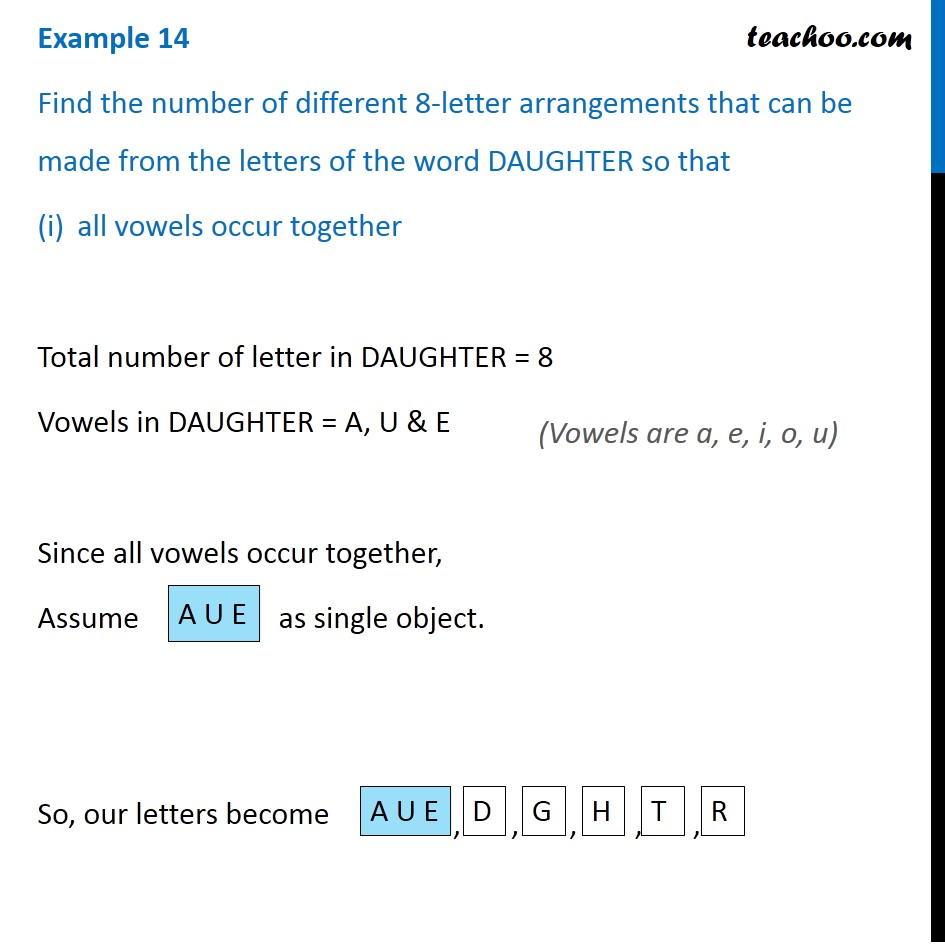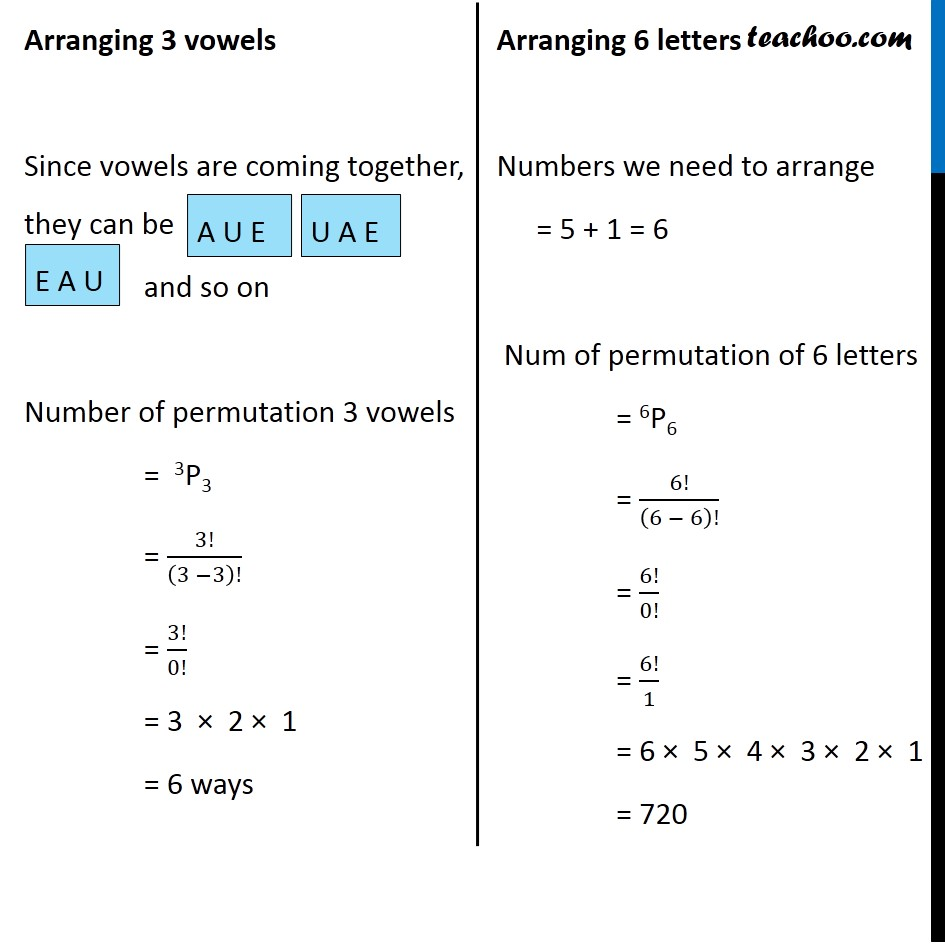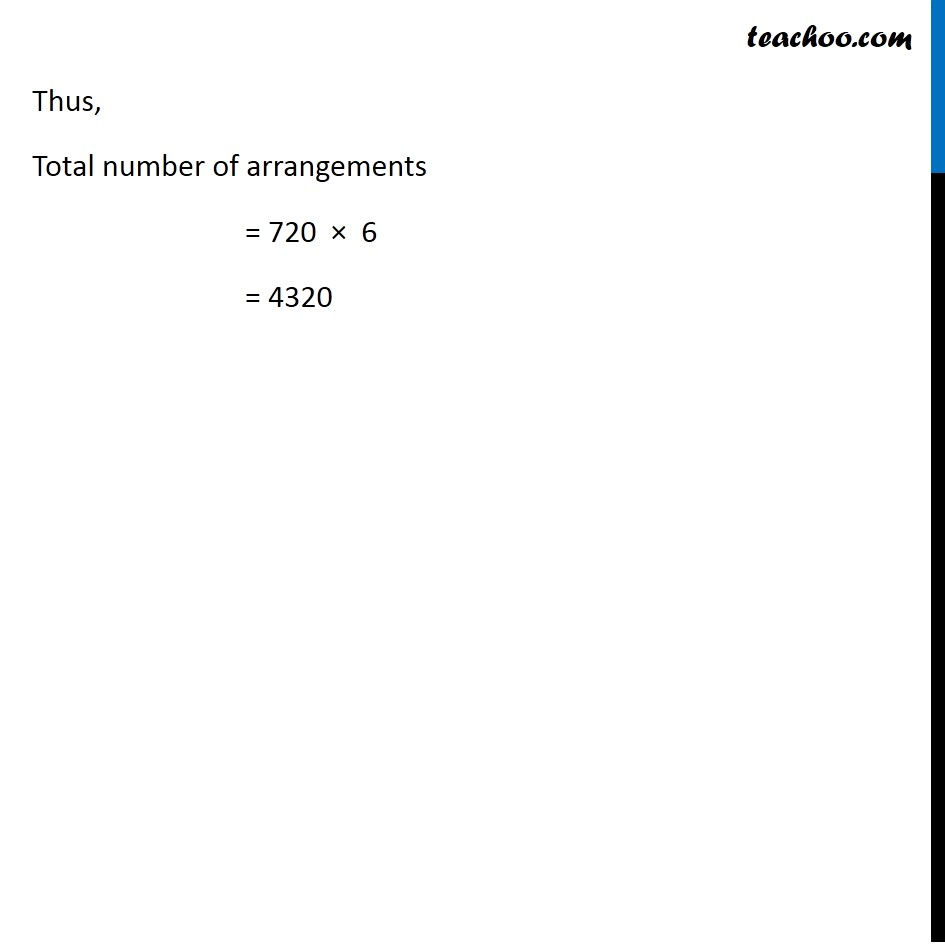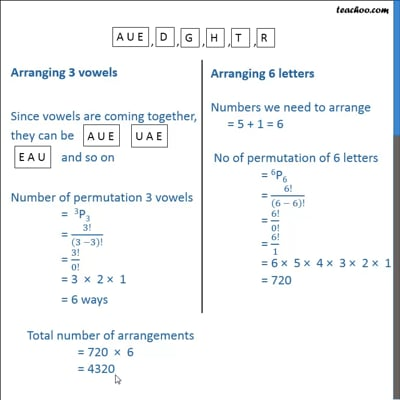This video is only available for Teachoo black users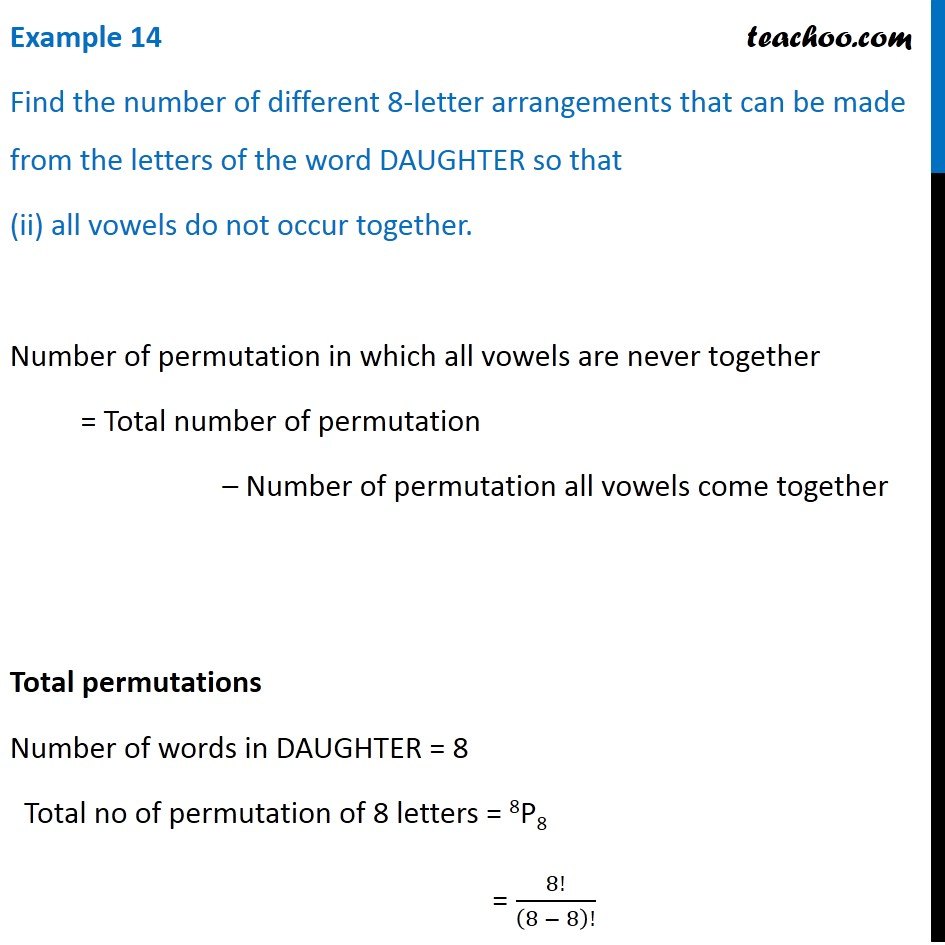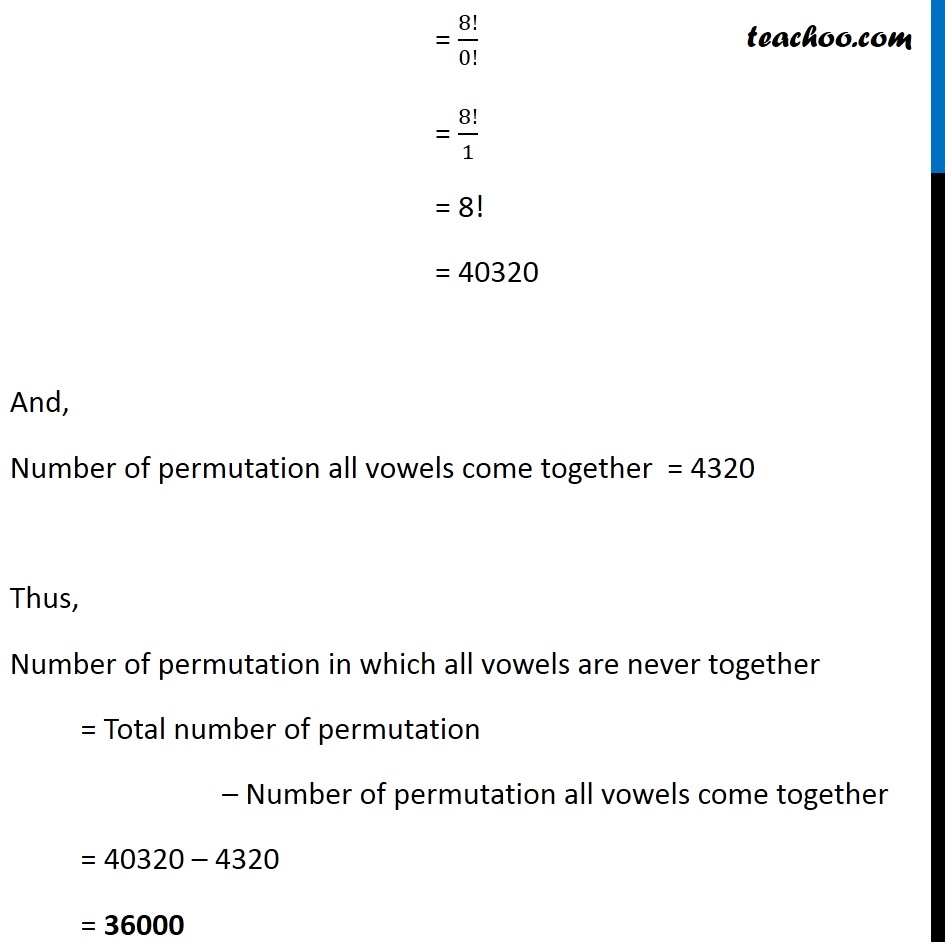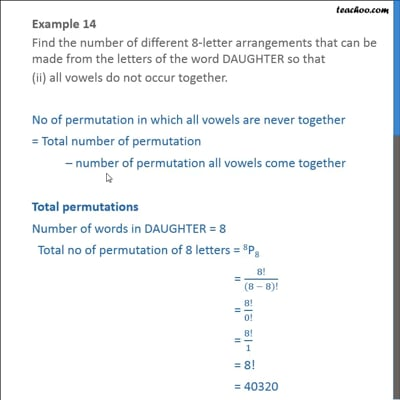This video is only available for Teachoo black users

### Transcript

Example 14 Find the number of different 8-letter arrangements that can be made from the letters of the word DAUGHTER so that all vowels occur together Total number of letter in DAUGHTER = 8 Vowels in DAUGHTER = A, U & E Since all vowels occur together, Assume as single object. So, our letters become (Vowels are a, e, i, o, u) Arranging 3 vowels Since vowels are coming together, they can be and so on Number of permutation 3 vowels = 3P3 = 3!/(3 −3)! = 3!/0! = 3 × 2 × 1 = 6 ways Arranging 6 letters Numbers we need to arrange = 5 + 1 = 6 Num of permutation of 6 letters = 6P6 = 6!/(6 − 6)! = 6!/0! = 6!/1 = 6 × 5 × 4 × 3 × 2 × 1 = 720 Thus, Total number of arrangements = 720 × 6 = 4320 Example 14 Find the number of different 8-letter arrangements that can be made from the letters of the word DAUGHTER so that (ii) all vowels do not occur together. Number of permutation in which all vowels are never together = Total number of permutation – Number of permutation all vowels come together Total permutations Number of words in DAUGHTER = 8 Total no of permutation of 8 letters = 8P8 = 8!/(8 − 8)! = 8!/0! = 8!/1 = 8! = 40320 And, Number of permutation all vowels come together = 4320 Thus, Number of permutation in which all vowels are never together = Total number of permutation – Number of permutation all vowels come together = 40320 – 4320 = 36000